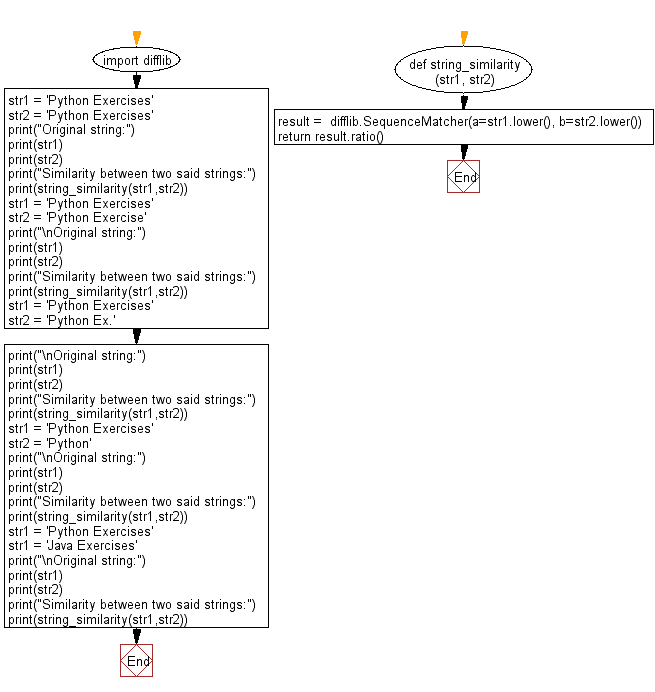﻿ Python: Find the string similarity between two given strings - w3resource# Python: Find the string similarity between two given strings

## Python String: Exercise-92 with Solution

Write a Python program to find the string similarity between two given strings.

From Wikipedia:
In computer science, approximate string matching (often colloquially referred to as fuzzy string searching) is the technique of finding strings that match a pattern approximately (rather than exactly).

Sample Solution:-

Python Code:

``````import difflib
def string_similarity(str1, str2):
result =  difflib.SequenceMatcher(a=str1.lower(), b=str2.lower())
return result.ratio()
str1 = 'Python Exercises'
str2 = 'Python Exercises'
print("Original string:")
print(str1)
print(str2)
print("Similarity between two said strings:")
print(string_similarity(str1,str2))
str1 = 'Python Exercises'
str2 = 'Python Exercise'
print("\nOriginal string:")
print(str1)
print(str2)
print("Similarity between two said strings:")
print(string_similarity(str1,str2))
str1 = 'Python Exercises'
str2 = 'Python Ex.'
print("\nOriginal string:")
print(str1)
print(str2)
print("Similarity between two said strings:")
print(string_similarity(str1,str2))
str1 = 'Python Exercises'
str2 = 'Python'
print("\nOriginal string:")
print(str1)
print(str2)
print("Similarity between two said strings:")
print(string_similarity(str1,str2))
str1 = 'Python Exercises'
str1 = 'Java Exercises'
print("\nOriginal string:")
print(str1)
print(str2)
print("Similarity between two said strings:")
print(string_similarity(str1,str2))
```
```

Sample Output:

```Original string:
Python Exercises
Python Exercises
Similarity between two said strings:
1.0

Original string:
Python Exercises
Python Exercise
Similarity between two said strings:
0.967741935483871

Original string:
Python Exercises
Python Ex.
Similarity between two said strings:
0.6923076923076923

Original string:
Python Exercises
Python
Similarity between two said strings:
0.5454545454545454

Original string:
Java Exercises
Python
Similarity between two said strings:
0.0
```

Flowchart:## Visualize Python code execution:

The following tool visualize what the computer is doing step-by-step as it executes the said program:

Python Code Editor:

Have another way to solve this solution? Contribute your code (and comments) through Disqus.

What is the difficulty level of this exercise?

Test your Programming skills with w3resource's quiz.

﻿

## Python: Tips of the Day

Check if a given key already exists in a dictionary:

In is the intended way to test for the existence of a key in a dict.

```d = {"key1": 10, "key2": 23}

if "key1" in d:
print("this will execute")

if "nonexistent key" in d:
print("this will not")
```

If you wanted a default, you can always use dict.get():

```d = dict()

for i in range(100):
key = i % 10
d[key] = d.get(key, 0) + 1
```

and if you wanted to always ensure a default value for any key you can either use dict.setdefault() repeatedly or defaultdict from the collections module, like so:

```from collections import defaultdict

d = defaultdict(int)

for i in range(100):
d[i % 10] += 1
```

but in general, the in keyword is the best way to do it.

Ref: https://bit.ly/2XPMRyz